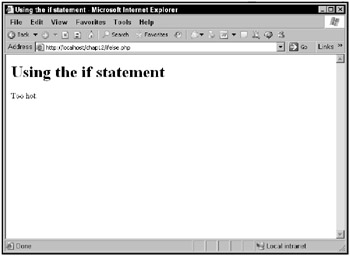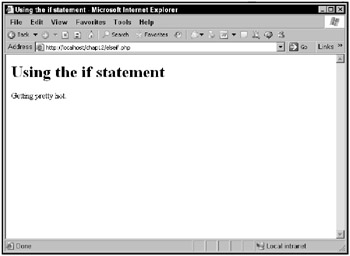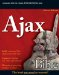Making Choices with the if Statement

Just as in JavaScript, PHP supports an if statement:

if (expression)    statement

As with JavaScript, in the previous code, expression is a true/false expression. If it evaluates to true,statement (which is usually enclosed in curly braces) is executed; otherwise, statement is not executed.

Here’s an example. The following code checks \$temperature to make sure it’s below 80 degrees, and since it is, displays the text "Not too hot.":

<html>     <head>         <title>             Using the if statement         </title>     </head>     <body>         <h1>             Using the if statement         </h1>         <?           \$temperature = 75;           if (\$temperature < 80)             echo "Not too hot.";         ?>     </body> </html>

What if you want to execute multiple statements? You enclose them in curly braces, { and }:

<html>     <head>         <title>             Using the if statement         </title>     </head>     <body>         <h1>             Using the if statement         </h1>         <?           \$temperature = 75;           if (\$temperature < 80){             echo "Not ";             echo "too ";             echo "hot.";           }         ?>     </body> </html>

As you can see, you can use comparison operators like < in if statements. Table 12.2 lists all the available PHP conditional operators.

Table 12.2: The Comparison OperatorsOpen table as spreadsheet

Operator

Operation

Example

Result

==

Equal

\$a==\$b

true if \$a is equal to \$b

===

Identical

\$a===\$b

true if \$a is equal to \$b, and they are of the same type

!=

Not equal

\$a!=\$b

true if \$a is not equal to \$b

<>

Not equal

\$a<>\$b

true if \$a is not equal to \$b

!==

Not identical

\$a!==\$b

true if \$a is not equal to \$b, or they are not of the same type

<

Less than

\$a<\$b

true if \$a is less than \$b

>

Greater than

\$a>\$b

true if \$a is greater than \$b

<=

Less than or equal to

\$a<=\$b

true if \$a is less than or equal to \$b

>=

Greater than or equal to

\$a>=\$b

true if \$a is greater than or equal to \$b

For example, if you wanted to check whether the value in the variable \$temperature was exactly 75 degrees, you could use the equality operator (==):

<html>    <head>        <title>            Using the if statement        </title>    </head>        <body>        <h1>            Using the if statement        </h1>        <?          \$temperature = 75;          if (\$temperature == 75){            echo "Not ";            echo "too ";            echo "hot.";          }        ?>    </body> </html>

As with JavaScript, you can also use logical operators to connect the conditions you test. For example, if you wanted to make sure the temperature was between 70 and 80, you could do this in your code:

<html>     <head>         <title>             Using the if statement         </title>     </head>     <body>         <h1>             Using the if statement         </h1>         <?           \$temperature = 75;           if (\$temperature >= 70 && \$temperature <= 80){             echo "Not ";             echo "too ";             echo "hot.";           }         ?>     </body> </html>

Table 12.3 lists the logical operators available in PHP.

Table 12.3: The Logical OperatorsOpen table as spreadsheet

Operator

Operation

Example

Result

and

And

\$aand\$b

True if both \$a and \$b are true

or

Or

\$aor\$b

True if either \$a or \$b is true

xor

Xor

\$axor\$b

True if either \$a or \$b is true, but not both

!

Not

!\$a

True if \$a is not true

&&

And

\$a&&\$b

True if both \$a and \$b are true

||

Or

\$a||\$b

True if either \$a or \$b is true

Using else statements

You can also use else statements with if statements in PHP. An else statement contains code that will be executed if the if statement’s statement isn’t executed. For example, if the temperature is less than 80, you might display Not too hot, but if it’s 80 or above, you might display Too hot. Here’s what that looks like in code, in ifelse.php:

<html>    <head>        <title>            Using the if statement        </title>    </head>    <body>        <h1>            Using the if statement        </h1>        <?          \$temperature = 85;          if (\$temperature < 80){             echo "Not too hot.";                    }          else {            echo "Too hot.";          }        ?>    </body> </html>

The results are shown in Figure 12.10, where, as you can see, the weather’s too hot.Figure 12.10: Using if/else statements

Using elseif statements

There’s even more power built in to PHP if statements. You can also use elseif statements in PHP, something you can’t do in JavaScript. The elseif statement lets you test additional conditions -additional to the main condition in the if statement, that is-and execute code to match.

For example, what if you wanted to execute some code if the temperature was below 80, other code if the temperature was below 85, and so on? You could do that as in this code, elseif.php:

<html>     <head>         <title>             Using the elseif statement         </title>     </head>     <body>         <h1>             Using the elseif statement         </h1>         <?           \$temperature = 85;                                            if (\$temperature < 80){             echo "Not too hot.";           }           elseif (\$temperature < 85) {             echo "Still not too bad.";           }           elseif (\$temperature < 90) {             echo "Getting pretty hot.";           }            else {             echo "Definitely too hot.";           }         ?>     </body> </html>

You can see the results in Figure 12.11, which shows that it’s getting pretty hot.Figure 12.11: Using elseif statements

But what if you have dozens of conditions you want to test? It would get a little tedious to test them all, one by one, with elseif statements. That’s why the switch statement exists.Ajax Bible
ISBN: 0470102632
EAN: 2147483647
Year: 2004
Pages: 169

Similar book on Amazon